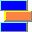# Erlang B Queueing Model (M/M/n/n)

For general concepts of queueing theory that apply to this queueing model, see About Queueing Models.

## What is the Erlang B Queueing Model?

In the Erlang B queueing model, customers arrive at a queueing system having n servers but having 0 waiting positions.  We assume Poisson arrivals and exponentially distributed service times.  Therefore, in Kendall notation, this model is denoted M/M/n/n.  Since there are no waiting positions, it is understood that if a customer finds upon arrival that no servers are available, then the customer goes away and is lost.  For this reason, the model is called a loss system.  These lost customers are also said to experience blockage or to be blocked.

## The Erlang B Function

The function B(n, x) defined by

B(n, x) =  (xn/n!) / (1 + x + x2/2! + x3/3! + ... + xn/n!)

computes the probability that an arriving customer will be blocked in the Erlang B model.  Mathematicians usually call this function the Erlang Loss Function, but in this help file, we will call it the Erlang B function.  It is an important building block in computational queueing theory.  In fact, the implementations of most functions in the Erlang Library for Excel involve computing the Erlang B function at least once, and sometimes many times.  The Erlang B function itself occurs in the Erlang Library for Excel under the name ErlbBlockage.

## Definitions and Formulas

### Erlang B Formulas

n = number of servers

λ = Arrival rate

μ = Service rate

x = Traffic intensity measured in erlangs

B = Probability of blockage
= Average fraction of callers that are blocked
See ErlbBlockage.

AHT = Average Handle Time (average duration of service)

NB = Average Number of Busy servers

NS = Average Number of customers in System

UTIL = Utilization fraction (occupancy, average fraction of time that each server is busy)

x = λ/μ

B = B(n,x)  (Erlang B function. See above)

AHT = 1/μ

NB = (1 − B)*x

NS = NB
= (1 − B)*x

UTIL = NB/n
= (1 − B)*x/n

In these formulas, the quantities B, AHT, etc., are theoretical averages that are approached as a limit under the assumption that the queue operates for a very long period of time without any change to the queue parameters (number of servers, arrival rate, service rate).

## Telecom Application

Analysts often use the Erlang B queueing model to describe the behavior of a group of incoming trunks in a pbx or telephone exchange.  As in any application of queueing theory, there are three parts that fit together: (1) a queueing model, (2) a real-world system, and a (3) mapping of the queueing model to the real-world system (see About Queueing Models).  In this example, the queueing model is Erlang B, and the real-world system is a group of trunks.  The mapping of the queueing model to the real-world system looks like this:

 Erlang B Entity Trunk Group Entity Server Trunk Customer Caller Arrival rate Calls arriving per second Average Handle Time Average Trunk Hold Time for calls Blocked customer Caller who gets a busy signal (all trunks busy)

Generations of telephone engineers have succesfully used the Erlang B model to predict blockage in telephone trunk groups, despite the fact that no telephone trunk group exactly matches the assumptions of Erlang B.  Perhaps the most questionable assumption is that when a caller is blocked (i.e., finds all trunks busy), the customer then goes away, never to return.  If a lot of blocked customers start a cycle of re-calling in order try to get through, then the assumption of Poisson arrivals will fail.  (Agner Erlang himself dealt with re-calling by using the Erlang C model.  He imagined that re-calling customers were placing themselves in a virtual waiting queue.)

Besides the study of blocking in trunk groups, there are many other applications of the Erlang B model—in telecom and in other fields as well.

Abstract Micro Systems, Nashville, Tennessee
Contact Abstract Micro Systems